Ex 8.1

Serial order wise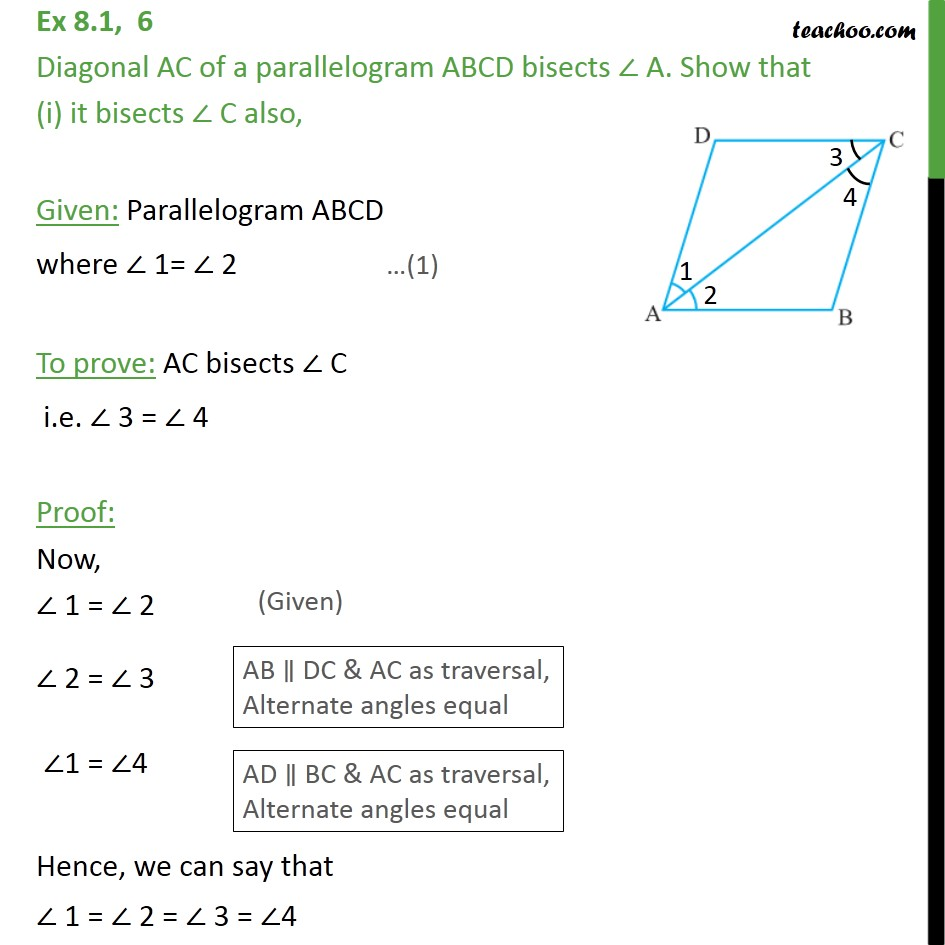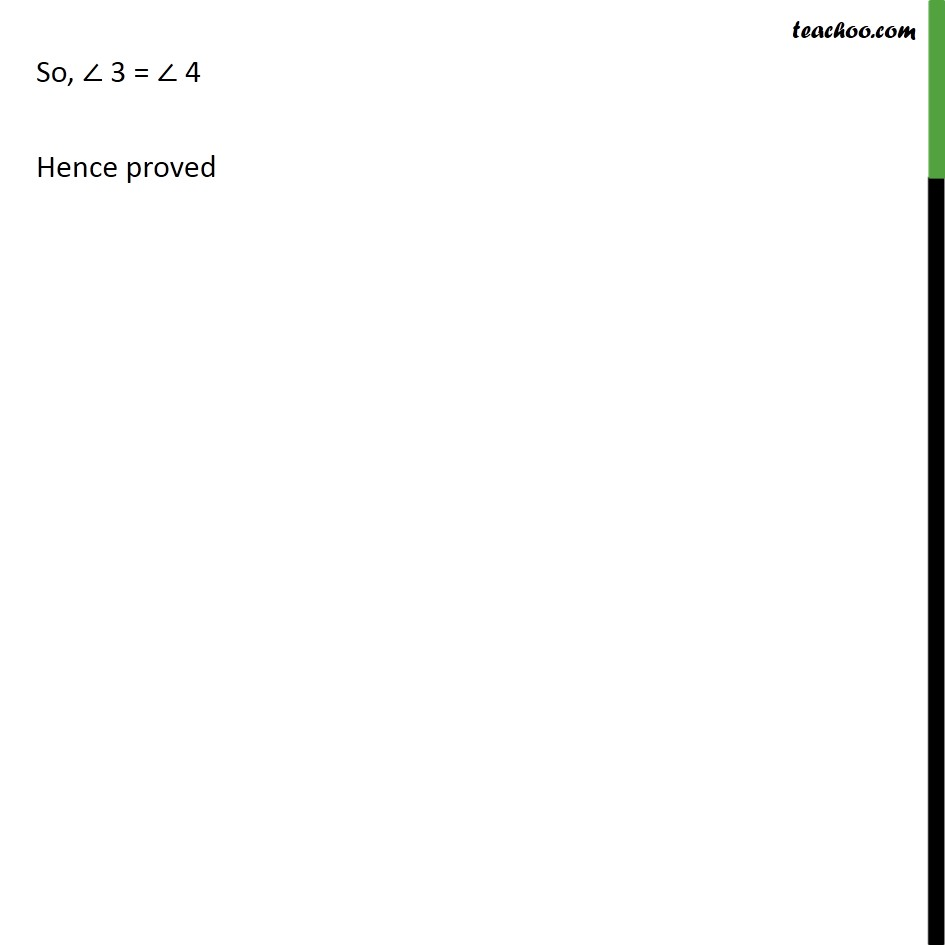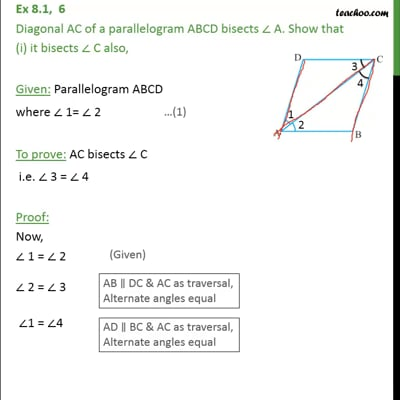This video is only available for Teachoo black users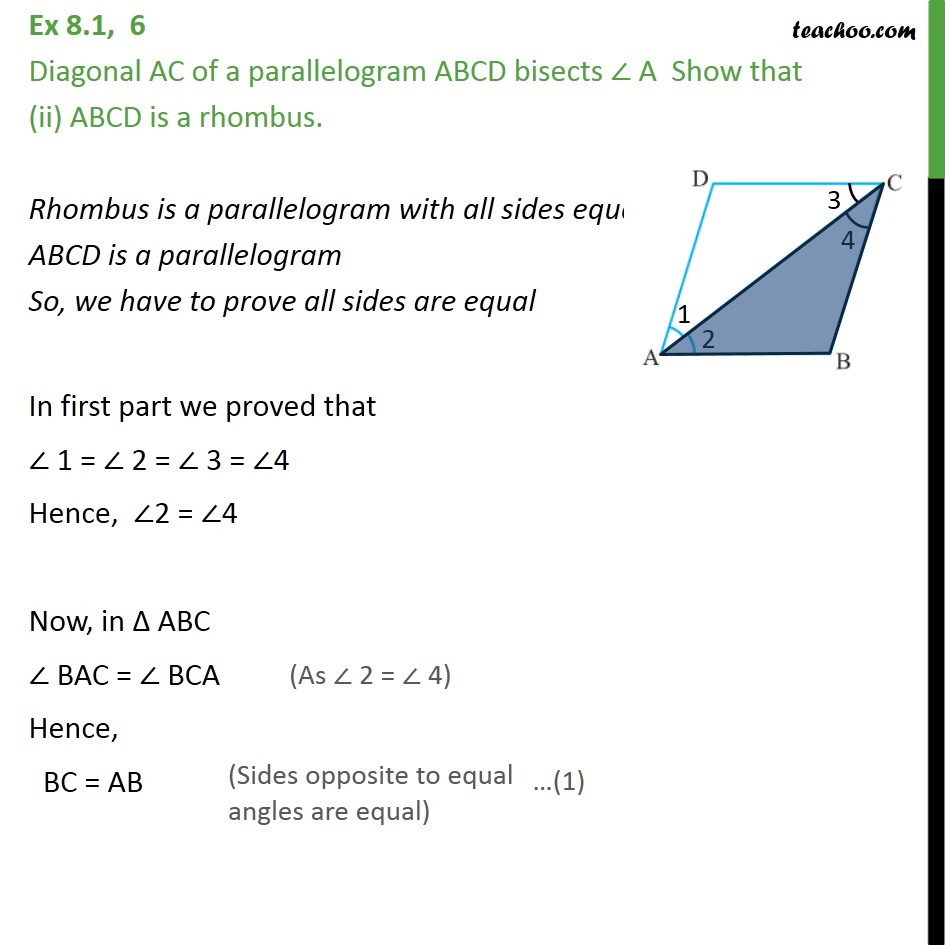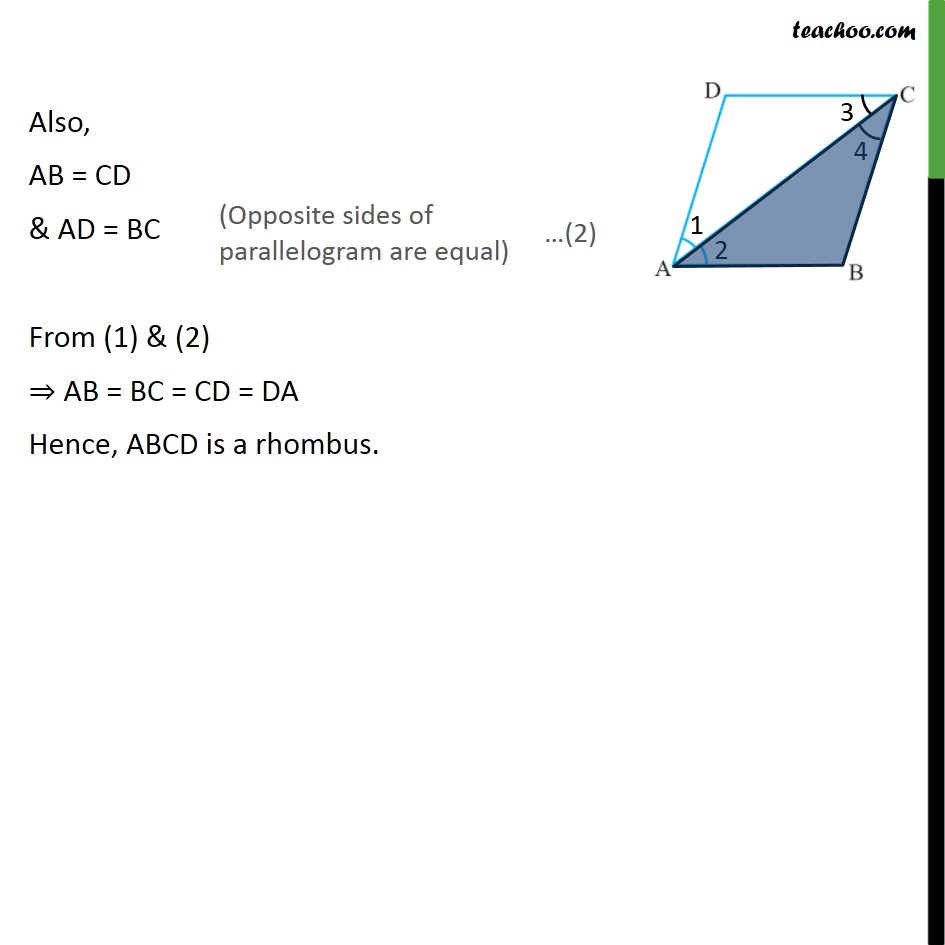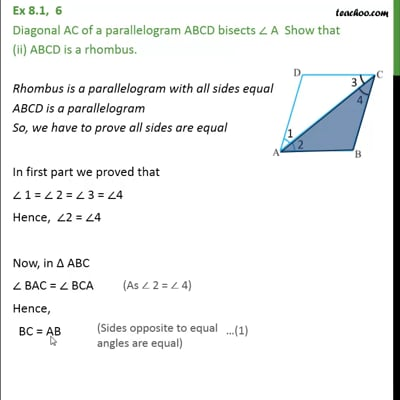This video is only available for Teachoo black users

Introducing your new favourite teacher - Teachoo Black, at only ₹83 per month

### Transcript

Ex 8.1, 6 Diagonal AC of a parallelogram ABCD bisects ∠ A. Show that (i) it bisects ∠ C also, Given: Parallelogram ABCD where ∠ 1= ∠ 2 To prove: AC bisects ∠ C i.e. ∠ 3 = ∠ 4 Proof: Now, ∠ 1 = ∠ 2 ∠ 2 = ∠ 3 ∠1 = ∠4 Hence, we can say that ∠ 1 = ∠ 2 = ∠ 3 = ∠4 So, ∠ 3 = ∠ 4 Hence proved Ex 8.1, 6 Diagonal AC of a parallelogram ABCD bisects ∠ A Show that (ii) ABCD is a rhombus. Rhombus is a parallelogram with all sides equal ABCD is a parallelogram So, we have to prove all sides are equal In first part we proved that ∠ 1 = ∠ 2 = ∠ 3 = ∠4 Hence, ∠2 = ∠4 Now, in Δ ABC ∠ BAC = ∠ BCA Hence, BC = AB Also, AB = CD & AD = BC From (1) & (2) ⇒ AB = BC = CD = DA Hence, ABCD is a rhombus.